Next: Nearest-neighbor coordinates Up: FAMILIAR OPERATORS Previous: Zero padding is the

Here we examine an example of the general idea that adjoints of products are reverse-ordered products of adjoints. For this example we use the Fourier transformation. No details of Fourier transformation are given here and we merely use it as an example of a square matrix.We denote the complex-conjugate transpose (or adjoint) matrix with a prime, i.e.,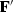.The adjoint arises naturally whenever we consider energy. The statement that Fourier transforms conserve energy iswhere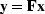.Substituting gives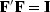, which shows that the inverse matrix to Fourier transform happens to be the complex conjugate of the transpose of.

With Fourier transforms, zero padding and truncation are especially prevalent. Most subroutines transform a dataset of length of 2n, whereas dataset lengths are often of length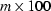.The practical approach is therefore to pad given data with zeros. Padding followed by Fourier transformationcan be expressed in matrix algebra as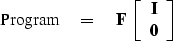(6)
According to matrix algebra, the transpose of a product, say,is the productin reverse order. So the adjoint subroutine is given by(7)
Thus the adjoint subroutine truncates the data after the inverse Fourier transform. This concrete example illustrates that common sense often represents the mathematical abstraction that adjoints of products are reverse-ordered products of adjoints. It is also nice to see a formal mathematical notation for a practical necessity. Making an approximation need not lead to collapse of all precise analysis.Next: Nearest-neighbor coordinates Up: FAMILIAR OPERATORS Previous: Zero padding is the
Stanford Exploration Project
12/26/2000